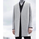574 views
// An example of binary options signals with results - colored background of expiry candle. (green = WIN, red = LOSE)
// Arrows set for bearish and bullish harami candlestick patterns. (credits to repo32)
// Expiration set to 1 candle by default.
study(shorttitle="BOA", title="Binary Options Arrows (example) ", overlay=true)
// Inputs
E = input(1, minval=1, title="Expiry")
// Calculations
Bearish_harami = (close > open and open > close and open <= close and open <= close and open - close < close - open and open < open)
Bullish_harami = (open > close and close > open and close <= open and close <= open and close - open < open - close and open > open)
// Setups
CALL = Bullish_harami == 1
PUT = Bearish_harami == 1
ARROW = CALL - PUT
plotarrow(ARROW, colorup=lime, colordown=red, transp=0, minheight=10, maxheight=10)
// Results
WIN = (CALL==1 and close<close) or (PUT==1 and close>close)
LOSE = (CALL==1 and close>=close) or (PUT==1 and close<=close)
bgcolor(WIN==1 ? lime : LOSE==1 ? red : na, transp=70)